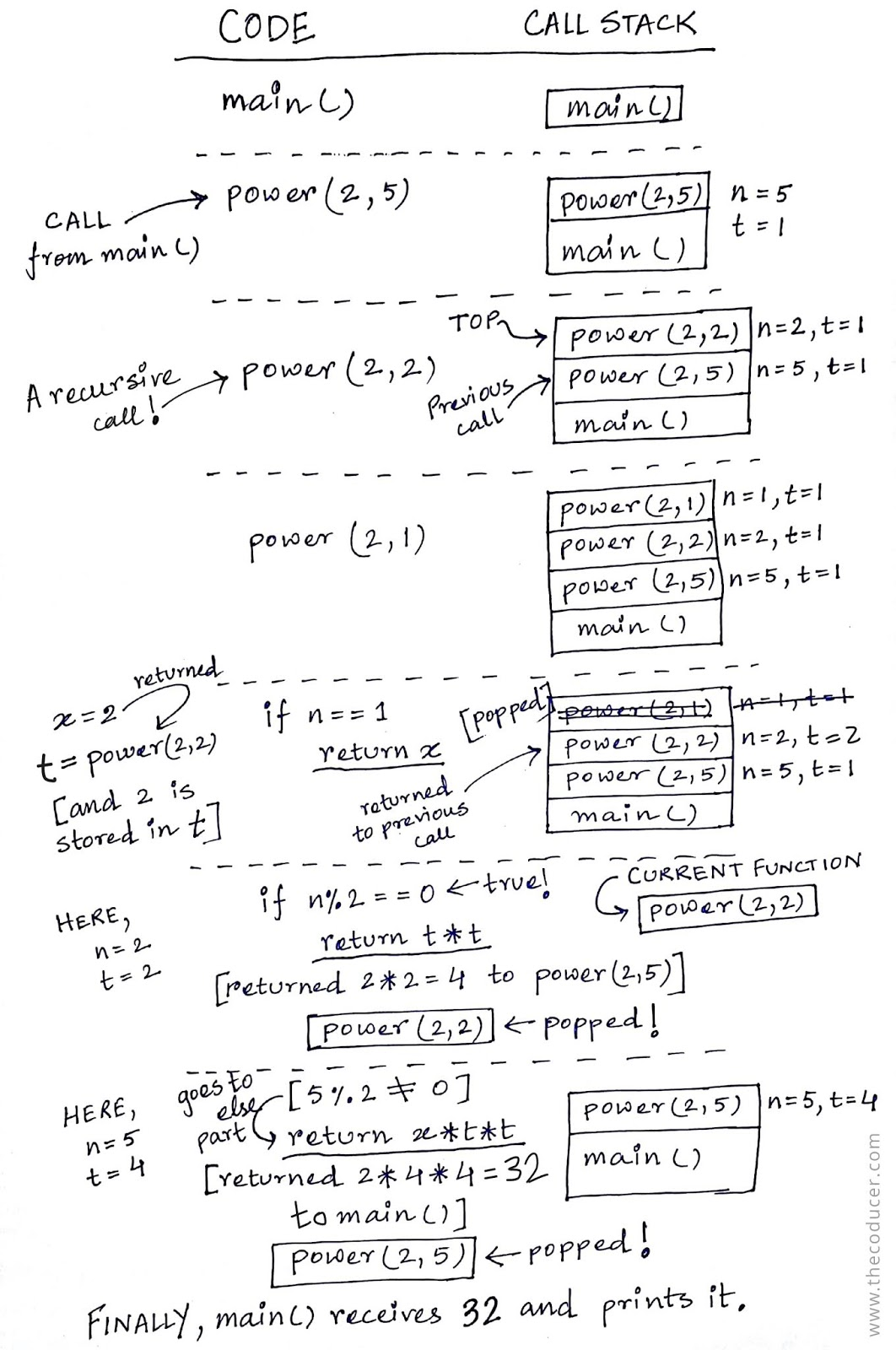### Compute the power of a number in O(log n) time

Computing the power of a number means to multiply a number $$x$$ by itself $$n$$ times. It can be written as $$x ^ n$$ where $$x$$ is called the base and $$n$$ is called the exponent. This mathematical operation is known as exponentiation.

Below is a simple program to compute the power of a number:
#include<stdio.h>
int main(void){
long x, n, r=1;
printf("Enter x and n: ");
scanf("%ld %ld", &x, &n);
printf("%ld ^ %ld ", x, n);
while(n--)
r = r * x;
printf("= %ld\n", r);
}

It is pretty evident that the time complexity of the above program is $$O(n)$$ since the while loop iterates exactly $$n$$ times. If we use divide and conquer approach then the complexity would get reduced to $$O(log\ n)$$.

Suppose, we want to calculate $$2^8$$ which could also be written as,
$(2^4)^2 = [(2^2)^2]^2 = [((2^1)^2)^2]^2 = 256$
It can be computed by using divide-and-conquer technique by dividing the $$n$$ by 2 until the value of $$n$$ becomes 1. This method is known as exponentiation by squaring.This is what divide-and-conquer does to $$2^8$$.

But if it's $$2^9$$ then we had to do, $$2 * 2^8$$. This is why in line no. 19 the $$x$$ is multiplied when the value of $$n$$ is not an even no. In the case of $$2^9$$ everything happens the same as in the calculation of $$2^8$$ until the $$n$$'s value comes down to 9 and the control goes to the else part and multiplies $$x$$.

Below is the code:
#include<stdio.h>
int main(void)
{
int x, n;
printf("Enter x and n: ");
scanf("%d %d", &x, &n);
printf("%d ^ %d = %d", x, n, power(x, n));
return 0;
}
int power(int x, int n){
int t=1;
if(n==1){
return x;
}
t = power(x, n/2);
if((n%2)==0){
return(t * t);
}else{
return(x * t * t);
}
}


The working of this program may not be very clear to you. So, I made an illustration (Ignore my drawing skills. It's really bad!) to help you understand how the recursion is working. Like normal function calls, recursion uses something called a call stack. Starting from the main(), each and every function call is pushed to the stack and are popped only when the current function(the one who is at the top of the stack) finishes its task or when it encounters a return statement.Illustration for $$2^5$$, where $$x$$ is 2 and $$n$$ is 5

I hope this article helped you in understanding the efficient $$O(log\ n)$$ solution for computing the power of a number and in getting a clear picture of how the recursion worked. I would like to recommend you all that whenever you're trying to learn new stuff and writing code, always try to break down everything to very basics and understand those little pieces first. This way you'll gradually get to see the bigger picture.

If you've found any errors or want to give a feedback then comment below.